Manfred MOHRLaserglyphs (1990-1993)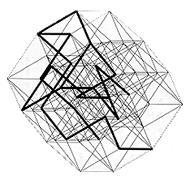This work phase (1991-92) is based on the 6-dimensional hyper-cube. This geometrically defined structure has 32 diagonals. The two endpoints of each diagonal lie diametrically opposite in the structure. A "diagonal-path" is the connection of two such diametric points through the network of edges of this complex structure. In a 6-D hyper-cube, each of these 32 diagonals have 720 different "diagonal-paths".

For each "Laserglyph" a random selection of four "diagonal-paths" from this repertoire of 23040 (32x720) possible paths is made. The 2-D dimensional projection of such groups of four are cut out of a metal plate by a laser to form a relief.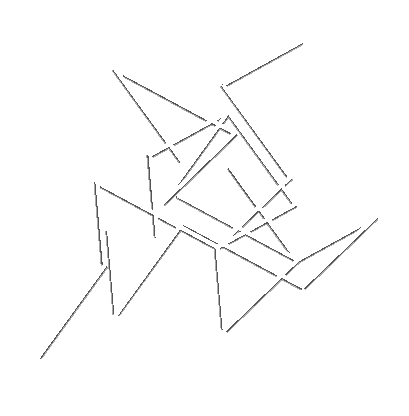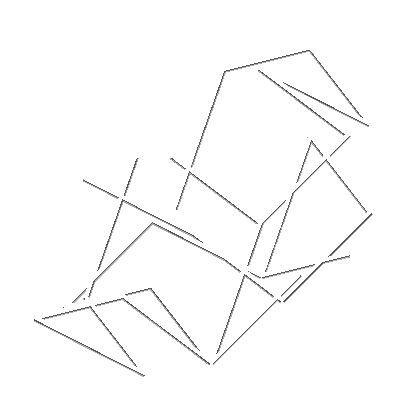P-486/O P-486/S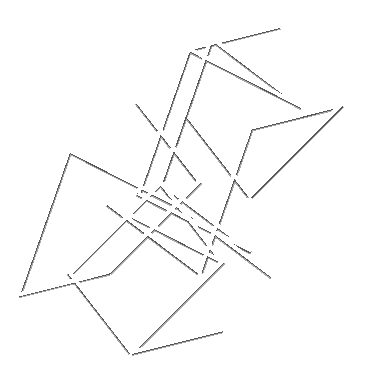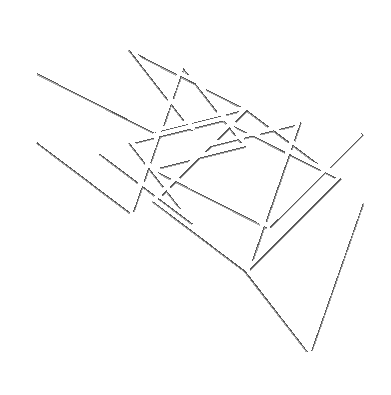P-486/V P-486/T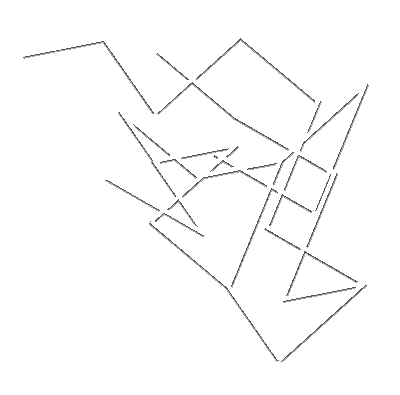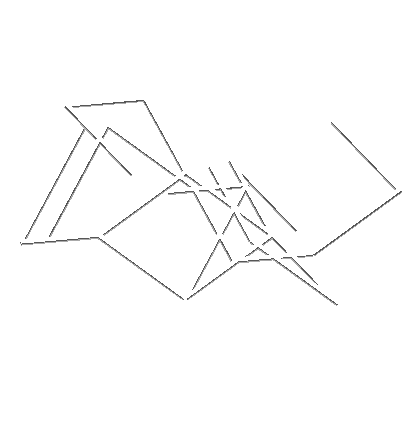P-486/K P-487/T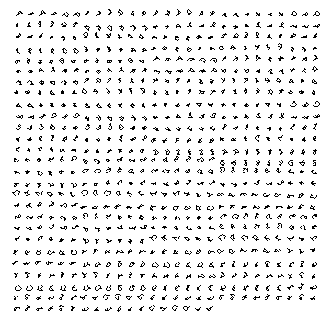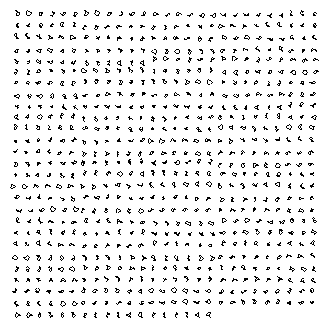P-480/110010 P-480/111000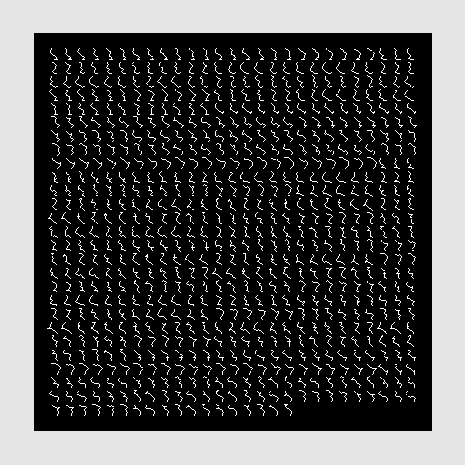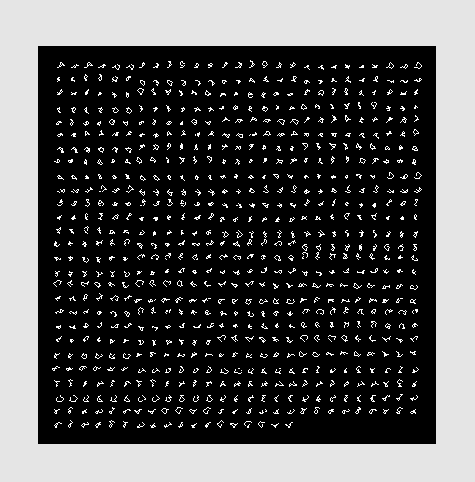P-480/001001 P-480/110010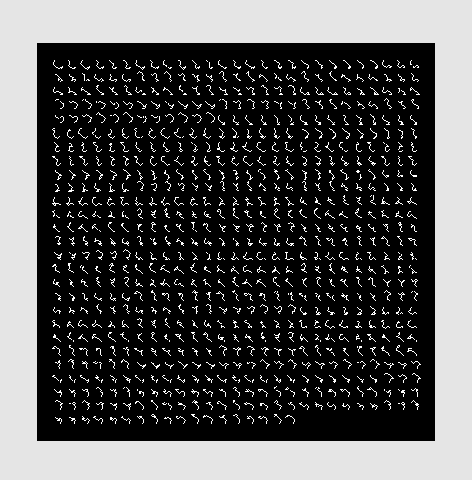P-480/101011